# Algebra 1 : Decimals and Percentage

## Example Questions

### Example Question #3011 : Algebra 1

If Sally cuts 50% of an apple for a pie, what is the decimal equivalence for what has been put into the pie?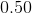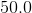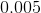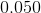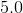Explanation:

This problem is simply asking us to convert 50% into its decimal equivalence.

The quickest way to switch between decimals and percentage is by keeping in mind that it is a factor of 100. For instance, decimals may be converted to percents by multiplying by 100 whereas turning percents to decimals would be the opposite operation (division). This is true because by definition, a percentage is a portion of 100. In this case, we are interested in a portion of 50.

Given this information,

we may convert from percent to decimal. This would require dividing by 100.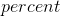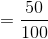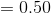### Example Question #3011 : Algebra 1

What is the decimal equivalence of 46%?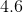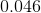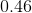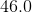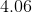Explanation:

This problem is simply asking us to convert 46% into its decimal equivalence.

The quickest way to switch between decimals and percentage is by keeping in mind that it is a factor of 100. For instance, decimals may be converted to percents by multiplying by 100 whereas turning percents to decimals would be the opposite operation (division). This is true because by definition, a percentage is a portion of 100. In this case, we are interested in a portion of 46.

Given this information,

we may convert from percent to decimal. This would require dividing by 100.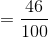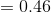### Example Question #81 : Decimals And Percentage

Find the decimal equivalent of the percent value of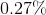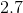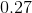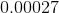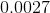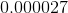Explanation:

To get a decimal value of a percent, we must divide the percent value by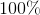: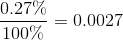### Example Question #82 : Decimals And Percentage

Which of the following is the decimal equivalent of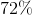?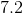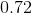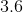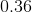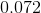Explanation:

To find the decimal equivalent of a percentage, simply move the decimal (if there is none, remember that there is always an implied decimal after the one's digit) to the left twice.

In other words divide the percentage by one hundred.

Thus the answer is: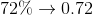or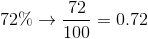### Example Question #81 : Decimals And Percentage

Which of the following is the decimal equivalent of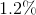?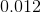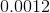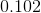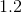Explanation:

To find the decimal equivalent of a percentage, simply move the decimal (if there is none, remember that there is always an implied decimal after the one's digit) to the left twice. In other words, divide the percentage by one hundred.

Thus, the answer is: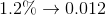or by dividing by one hundred. Then multiply the numerator by ten to eliminate the decimal. Once the decimal is eliminated, perform division as usual.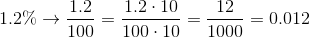### Example Question #3014 : Algebra 1

If you owe the bankof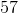dollars, how much money do you owe them?

Round your answer to the nearest cent.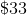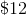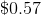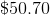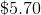Explanation:

To solve this problem, first, you must convert the percentage above into a decimal.

To find the decimal equivalent of a percentage, simply move the decimal (if there is none, remember that there is always an implied decimal after the one's digit) to the left twice. In other words divide the percentage by one hundred.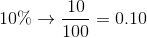Then, you take your result and multiply it by the initial amount for your answer: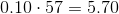### Example Question #3015 : Algebra 1

If you owe the bank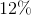ofdollars, how much money do you owe them?

Round your answer to the nearest cent.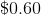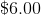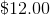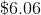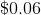Explanation:

To solve this problem, first, you must convert the percentage above into a decimal. To find the decimal equivalent of a percentage, simply move the decimal (if there is none, remember that there is always an implied decimal after the one's digit) to the left twice. In other words, divide the percentage by one hundred.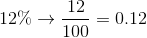Then, you take your result and multiply it by the initial amount for your answer: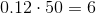### Example Question #83 : Decimals And Percentage

Write the following percentage as a decimal.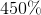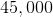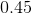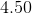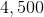Explanation:

Write the following percentage as a decimal.To write a percentage as a decimal, we simply need to move the decimal place two spaces to the left.

Thus,Becomes,### Example Question #3011 : Algebra 1

Find the decimal of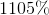.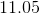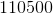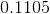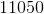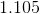Explanation:

The number in percent is out of 100 parts.  Remove the percentage sign and divide this number by 100.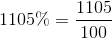This is the same as moving the decimal place of 1105 two places to the left.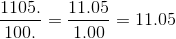The answer is:### Example Question #81 : Decimals And Percentage

Find the decimal of.Explanation:

The number in percent is out of 100 parts.  Remove the percentage sign and divide this number by 100.This is the same as moving the decimal place of 1105 two places to the left.The answer is:### All Algebra 1 Resources